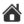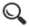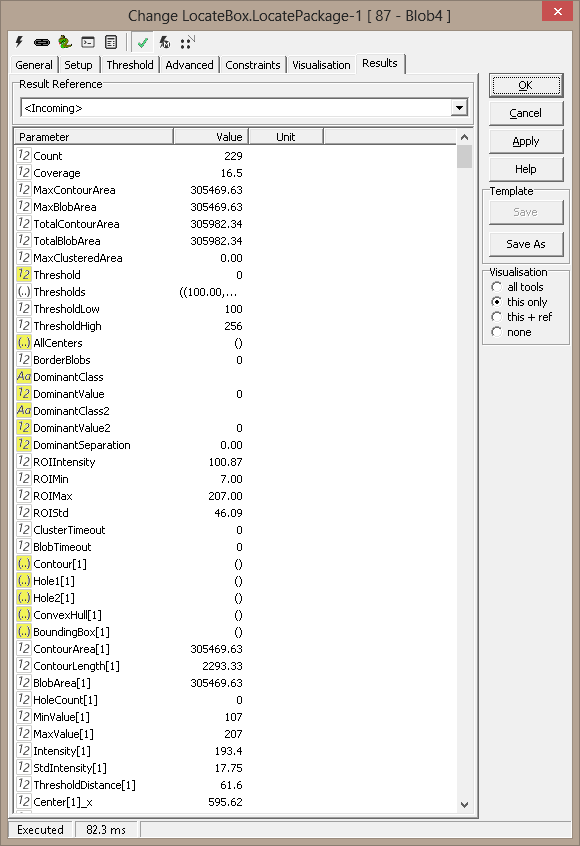Up | Setup | Threshold | Advanced | Constraints | Visualisation | Results | Commands | Shortcuts | Examples | Release NotesResults
 Count Number of blobs found Coverage Total blob area in percent of the ROI-area Largest contour area Largest contour area (hole area included). Largest blob area Largest blob area (hole area subtracted). Total contour area Total area of all blobs (hole area included). Total blob area Total area of all blobs (hole area subtracted). Threshold Threshold calculated by Auto or Percentile Thresholds All thresholds (as a Python tuple), also multipass Threshold low Low threshold, specified or calculated by Percentiles Threshold high High threshold, specified or calculated by Percentiles All centers Python tuple with sorted blob centers of gravity Dominant class Feature classification: dominant class name. Dominant value Feature classification: largest value Second dominant class Feature classification: next dominant class name Second dominant value Feature classification: second largest value Dominant separation Feature classification: separation between these values ROI intensity Statistics for ROI (not calculated if specified thresholds) ROI min Statistics for ROI (not calculated if specified thresholds) ROI max Statistics for ROI (not calculated if specified thresholds) ROI std Statistics for ROI (not calculated if specified thresholds) Cluster timeout 1 if clustering has timed out (no clustering performed) Contour[n] Contour polygon for blob n Hole1[n] Contour polygon for largest hole in blob n Hole2[n] Contour polygon for second largest hole in blob n Convex hull[n] Convex hull polygon for blob n Bounding box[n] Bounding box polygon for blob n Contour area[n] Contour area for blob n Contour length[n] Contour length for blob n Blob area[n] Area (ex. holes) for blob n No of holes[n] Number of distinct holes inside blob n Intensity[n] Average intensity for blob n Std intensity[n] Intensity variation for blob n Threshold distance[n] Distance to blob threshold Center[n].x x position of blob center Center[n].y y position of blob center CenterDistance[n] Distance from blob center to origin Major axis[n] Length of blob major axis Minor axis[n] Length of blob major axis Circularity[n] Blob shape (1=perfect circle) Axis ratio[n] Major axis / minor axis Angle[n] Angle of major axis Length[n] Bounding box length Width[n] Bounding box width Bounding box area[n] Bounding box area Aligned length X[n] Axis aligned bounding box length Aligned width Y[n] Axis aligned bounding box width Aligned rect area[n] Axis aligned bounding box area Volume[n] Blob volume (area*intensity) Classification[n] Class name Distance[n] Distance to best match Separation[n] Separation best to second-best match Clustered[n] 1 if this is a blob clusterScorpion Vision Version XII : Build 646 - Date: 20170225 Scorpion Vision Software® is a registered trademark of Tordivel AS. Copyright © 2000 - 2017 Tordivel AS.